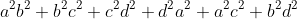## Saturday, 10 September 2011

### Determinants for M2 I: cyclic VS symmetrical

Recently I just heard someone saying that the factorization of determinants has homogeneous, cyclic and symmetrical properties. Now I'm going to clerify the above claim. In part 1 (this one), we will discuss the properties of function and in part 2 we will look at those determinants.

1) The definition of homogeneous is simple unique in this case. We have a unique factorization of a certain determinants, but why?

- The properties of determinants is based on the definition of the determinants.
- Each properties can be induced from definition.
- The properties are invertible.

Now if we are going to factorize S=Det(X), through properties X and Y respectively,
The expression through process X is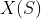while that of Y is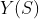, we have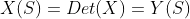.
The factorization result is equivalent each other, so the answer is homogeneous. If the expression is real number or real varaiable, by the fundamental theorem of algebra, it has an unique factorization over real number.

P.S. this is not a rigour proof on the homogeneous property of det., it just serves as a guide.

2) An expression is cyclic if a set of variable in a function f(a,b,c) = f(b,c,a) = f(c,a,b).
For example, let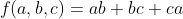,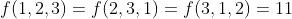, thus this is a cyclic function.

3) An expression is symmetrical if a set of variable in a function that the order of variable can interchange while the value of function is unchanged. For example, let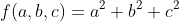, then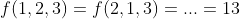.

4) A symmetrical function must be a cyclic function. This is obvious since symmetrical function permutes all varaible in the function in a random way but cyclic function changes their order in a specified way in which it can also be reached by symmetrical function.

5) A 2-varaible cyclic function must be symmetrical, because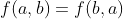is the only criteria to show that it's cyclic and symmetrical.

6)It's NOT the case that for more variable, cyclic is equivalent to symmetrical. Consider this function: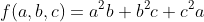, it's cyclic since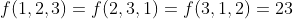, BUT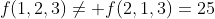.

7) We have some elemental function as the general form of composing a symmetrical function. Moreover, ALL symmetrical expression can be expressed in terms of sum and product of elemental function (if it's constant free)
Definition: elemental function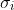of n variable is equal to the sum of all possible product of i-out-of-n distinct variable.
For example, 2 variable: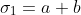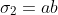3 variable: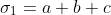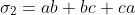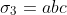Most probably you won't need to memorize the expression for higher degree.
For example, 2 variables:
1)2)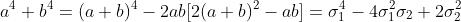3 variables:
1)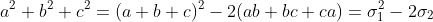2)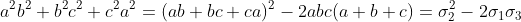8) Lastly this is a group of isolated function with the loss of beauty, asymmetrical function, means that if two neighbouring variables are interchanged, the value of function is multiplied by -1. The most most most most easy example: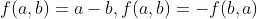Now that's all to give a basic definition for difference between cyclic and symmetrical functions.

Exercise:
1)Determine cyclicity/symmmetricity/asymmetricity of the following functions
a)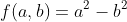b)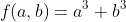c)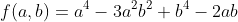d)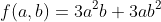e)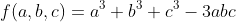f)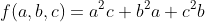g)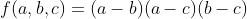h)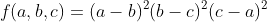i)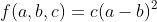2) a) Suggest a symmetrical functions for 3 variables
b) Suggest a cyclic and asymmetrical functions for 4 variables.
c) Suggest a function that is cyclic but neither symmetrical nor asymmetrical for 3 variables.
3) Can factorizing over complex number be unique? Explain Briefly.
4) Rewrite the following polynomials into sum and products:
a)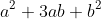b)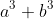c)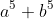d)e)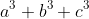f)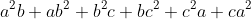g)